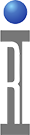Polynomial Fit to Measured Data

Measurement functions sometimes blur the lines between data collection and data analysis. In the case presented here, a measured data set will be used to fit a polynomial of third and fifth order, using a least squared error technique. The polynomial can easily be checked for fitness against the original ("training") data set, and the same polynomial can be used with measurements other than the set used to create (or "train") it. These functions use software available in the Cassini test platform and are not currently available in the RI7100A.

In the example testplan, the data comes from a CSV table of values. In general, the values will result from measurements done on a device. See the testplan panel below. The "X" and "Y" values represent independent and dependent variables, in this case a power vs. voltage measurement. This panel represents the measurements that will be used to create the polynomial.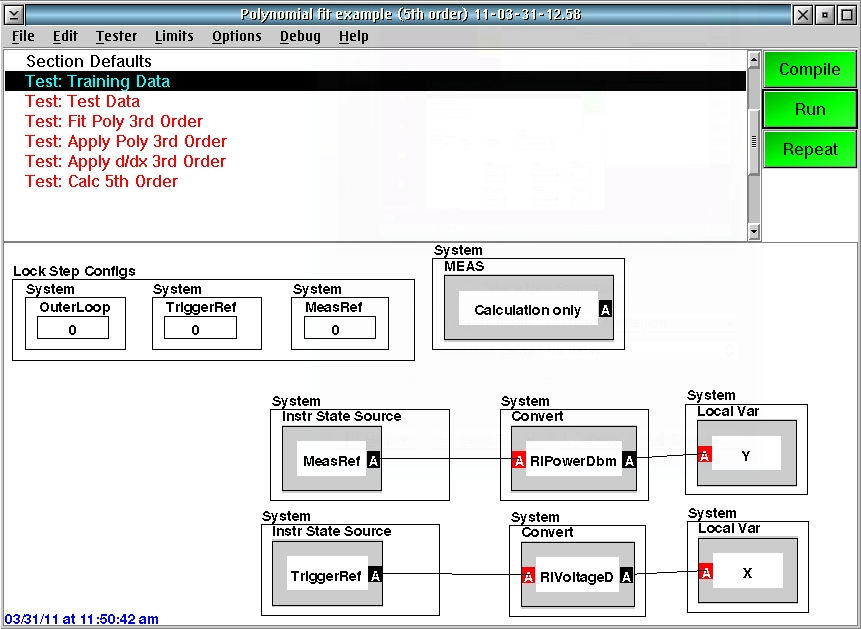One of the features of this application is that the polynomial may be used with data sets other than the one which was used for training. In this case, the independent variable, X, will be "measured" again and used with the polynomial to create an independent Y variable.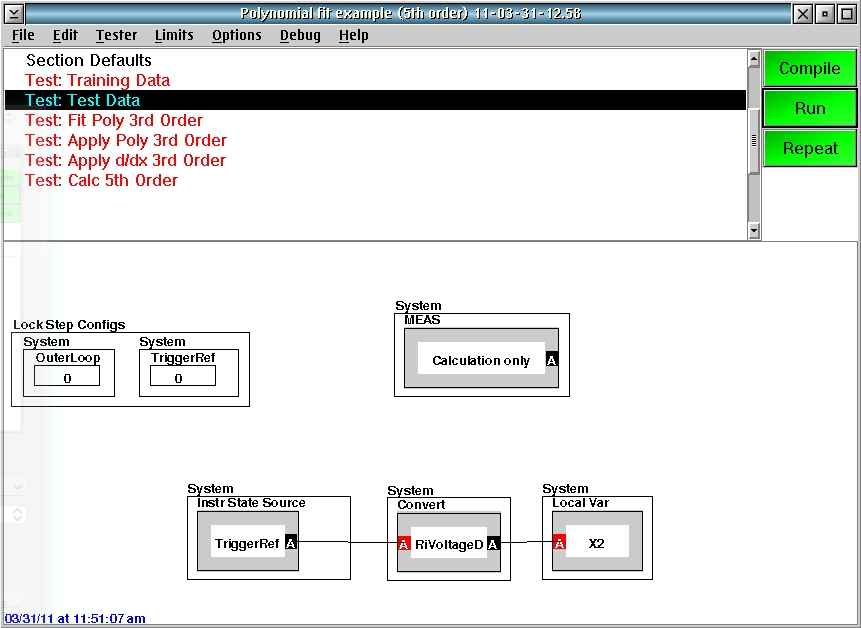The first step in the process, after creating the test data, is to fit the original data set (X and Y) to a third-order polynomial. This is accomplised by the panel shown below. Note the use of calculation "blocks" to access the new functions used in this application. This panel fits the training data to a polynomial and saves the resulting coefficients as "Coeff 3rd" in a local variable. The coefficients will be used later to create a new independent (Y) estimate. There is also support for differentiating the polynomial to provide the instantaneous slope of the independent Y variable vs. the dependent X variable, stored in "Coeff 3rd d/dx".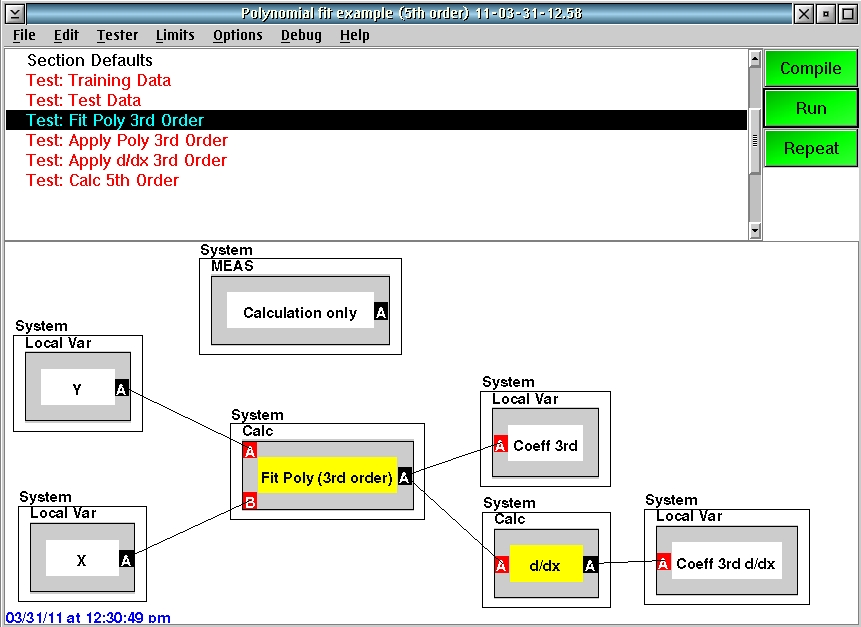Shown below is an example of the calculation used in the block "Fit Poly (3rd order)" The method named "polyThirdOrder" returns a set of basis functions used for the least-squares fit. There are two pre-defined basis function sets, polyThirdOrder and polyFifthOrder. Any set of orthogonal functions f(x) can be used in the least-squares fit.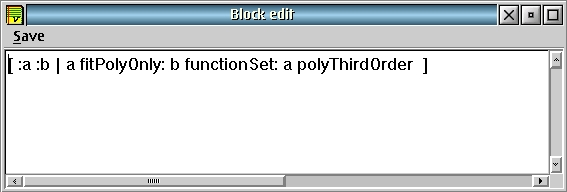There is a built-in differentiation function that expects the coefficients of a polynomial in X and returns the derivative as another function set. The contents of the block "d/dx" are shown below: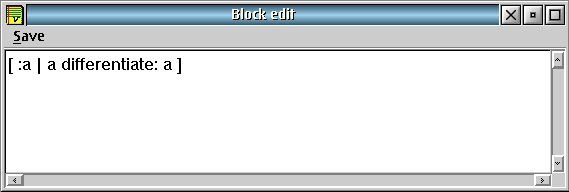After the polynomial has been created by the least-squares fit, it is applied to a data set as shown in the next panel. The polynomial is evaluated at a set of points defined by the "new" measurement, X2. (It could just as easily be evaluated with the training set, X.) The blocks labeled "Make XY" are only for ease of display. They turn the X and Y data into a "real1 vs. real2" data type that is more convenient to plot on the rectangular display of the Cassini tester. Calculations on this panel include the original training data ("Original"), the difference between the original independent data and the polynomial fit ("Delta 3rd") and the polynomial fit by itself ("Fit 3rd").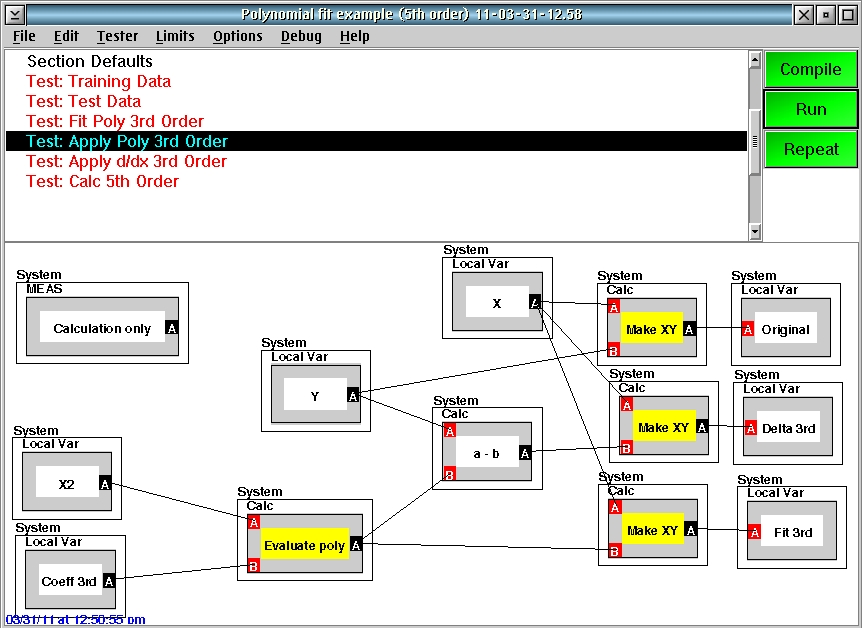The calculation block "Evaluate poly" is shown below. As before, the "polyThirdOrder" defines the function set used to interpolate the data. It MUST match the function set used to create the polynomial fit in the earlier test panel or a "size mismatch" error will result.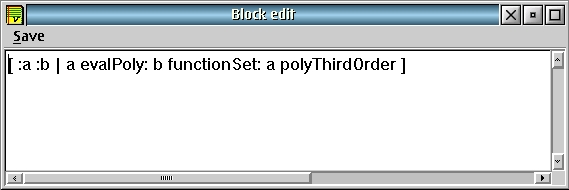The "Make XY" block is shown below. It creates a data variable of type RiReal1VsReal2 which is easier to display. It has no effect on the calculations and it is not needed in your testplan.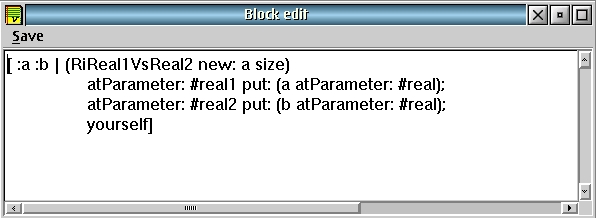Let us now see an example training data set and the results of the polynomial fit thus far. The original data set is plotted below. It is a plot of power vs. voltage. Note the horizontal axis is "real1" and the vertical Y axis is set to "real2" for this (and all other) plots in this note: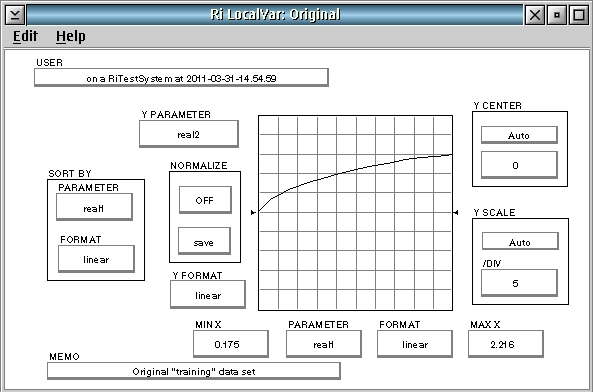The difference between the original training data and the polynomial applied to a new set of measurement points is shown below. This shows the flexibility of the calculation -- the polynomial can be created once and applied many times to different data sets.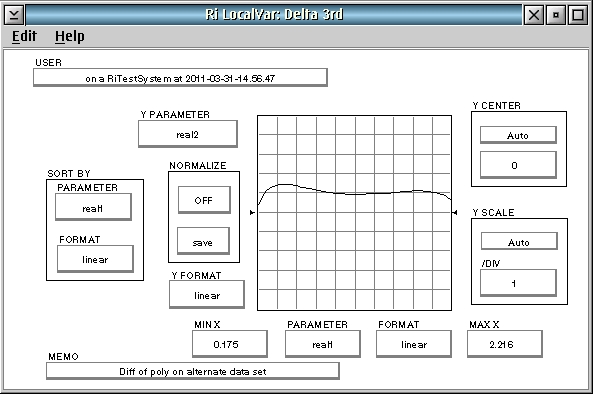To see how well the polynomial fits the original training data set, change the variable "X2" in the test panel above to "X" and re-run the testplan. The "Delta 3rd" plot now shows the closeness of fit between the training data set and the polynomial interpolation: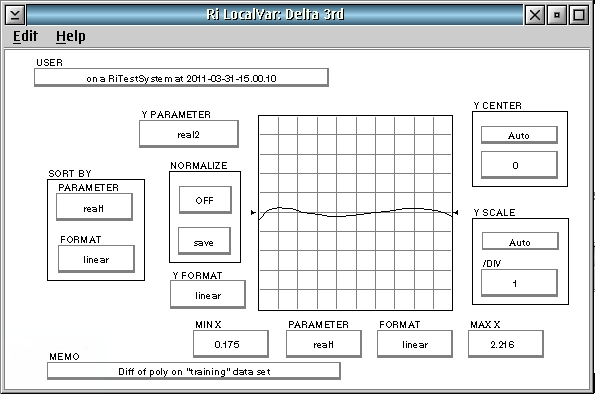The next test panel uses the derivative polynomial to plot the slope of the 3rd order poly at each of the independent variable points. The "Evaluate poly" and "Make XY" blocks are exactly as before.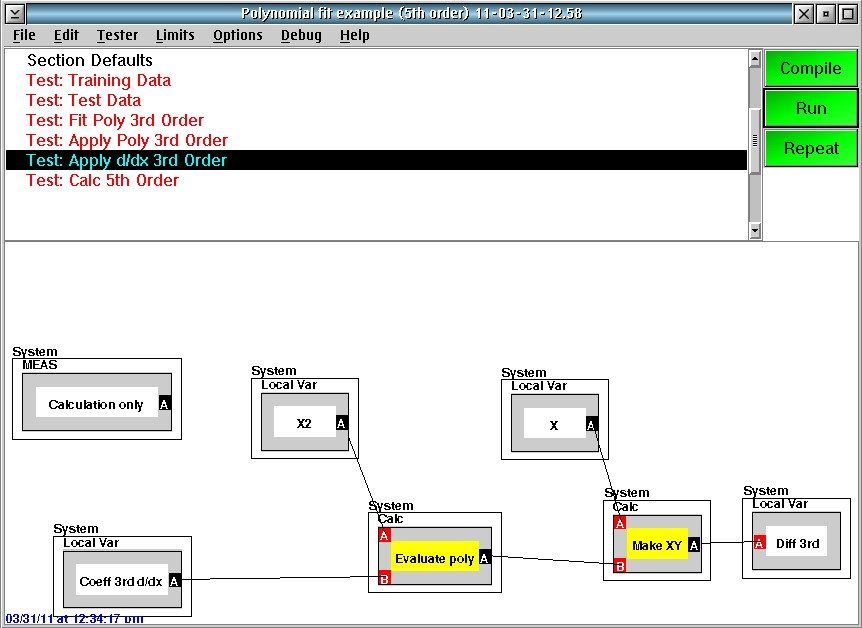The result of the slope of the poly fit is shown below. The slope is clearly a quadratic function, which makes sense since the original polynomial is cubic.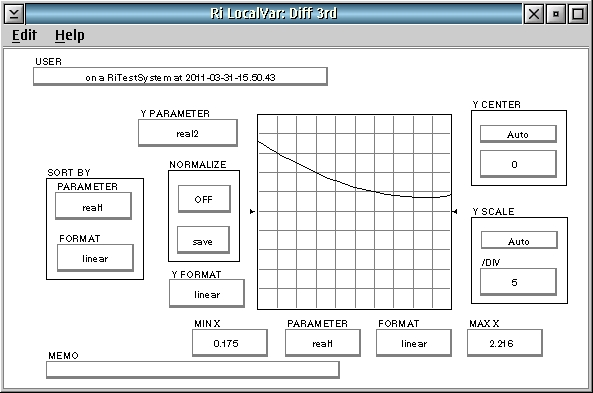As an exercise, the same polynomial fit is shown on one test panel for the 5th order case. As noted before, the only difference in the calculation blocks is the substitution of "polyFifthOrder" for "polyThirdOrder" to select the basis functions.To show the closeness of fit (to the original training data, not the alternate X2 data set), the variable "Delta" is plotted at high resolution -- 0.1/division.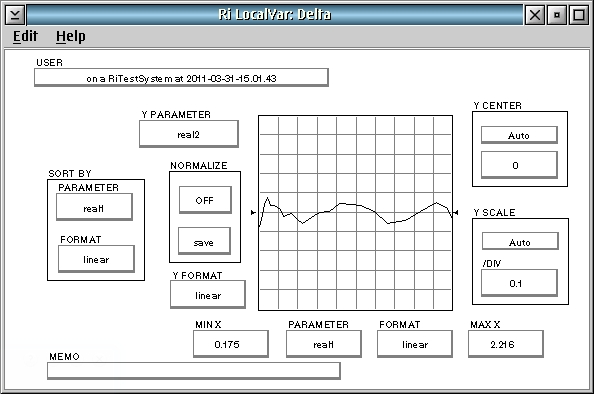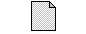PolyFit.gzp

https://roos.com/docs/JBAT-8FGTVD
ROOS INSTRUMENTS CONFIDENTIAL AND PROPRIETARY